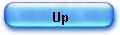HP 35 ex Algorithm

The algorithm used in HP35 for ex function is very simple to understand as long as the logarithm routine is known. To save explanations, I ask my readers to study first my previous articles (on Logarithms and trigonometry in the HP35’ ROM).

It is also derived from J.E. MEGGITT’s paper (Cf. page 220) (1).

The process (“Pseudo Division and Pseudo Multiplication Processes”) that leads to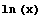can be reversed to form exponentials.

By definition we have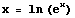; so if we can break the argument x in small quantities such as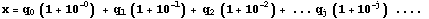and compute the pseudo quotients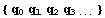, obviously we can reverse theprocess and compute: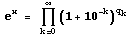by pseudo multiplication.

In fact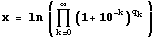is a simple application of logarithm definition.

As one can guess, the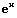algorithm uses the same routine in ROM as forand it was mandatory to use the same constants and the same accuracy level to assure symmetry. In certain cases it can be very tricky and, as a matter of fact, the operation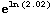(wrongly evaluated as 2) led HP to offer, in a rare elegance, to early 25000 owners, a replacement.

The routine starts at address 02010, with register C holding the argument (4.4 in my trace) in normalized form C=04400000000000 (remember it means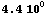, register A holding the number in displayable form and B holding the mask to display  B=02099999999999   meaning digit, point, digit, a “9” being blanked.

The order is reversed compared to; we load from ROM the constants: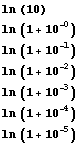and we compute by repetitive subtractions the pseudo quotients (as we have done in the first part of the tan(x) routine).

Let’s look in detail.

In [BP1] we start loading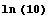running the same code as forand as we will do for the rest of the constants. At 02335 [BP2] the call to the normalization routine (nrm27 and nrm26) is just a trick to find a good “return” (the ROM room was rare).

At [BP3] there is a call to routine “pre21”, which is design to do a “prescaling” of the argument x (just like the trigonometric algorithm does with the angle): the user can enter any argument x and press the ex  so the “prescaling” routine is going to get back x to the 0 to 1 interval.

The routine performs repetitive subtraction offrom the argument, in our case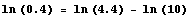can be made only one time to scale the argument to the requested interval.

To avoid to make too many subtractions for a big argument (say 44 for example) a simple trick is used:
we can subtract 19 times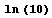from 44 but also one time from 4.4 then multiply the result by 10 (shift left) and again subtract 9 times; that’s exactly what the routine does, keeping track of the subtraction at different ranks. I’ve made a zoom on this part in another partial trace for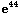.

At [BP4], prescaling is finished and we start to compute pseudo quotients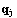in the logarithm’s reverse process: we load ln(2) using the same code as for logarithm ; it is done at address 02105, register C is holding ln(2) and is aligned with register A.

After shifting left A (at pqo11) to save accuracy, we try (in pqo16) to subtract a – b as many times as possible, pqo15 (c + 1 -> c[s]) keeps the count of loops in the sign byte of register C. This part is very simple and looks close to the code of trigonometry (part 2).

In my example of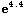the number of loops is 3 and this result is reached at [BP5], which leans that we could subtract 3 times from ln(2).

The same process is repeated for each constant and the final result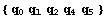is reached at [BP5], it is 2081031 and the scaling factor is 1 (this result is in register A).

As usual now, I will show the result calculated with Mathematica to ease understanding:

Repetitive subtractions= 2081031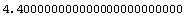- 1 * ln(10) =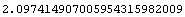1 - 3 * ln(2) =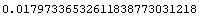3 - 0 * ln(1.1) =0 - 1 * ln(1.01) =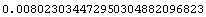1 - 8 * ln(1.001) =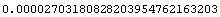8 - 0 * ln(1.0001) =0 - 2 ln(1.00001) =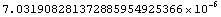2

the last line being the remainder after the subtractions cycle is completed.

The part2 of the calculation is using the routine “eca”, that we already met in the logarithm code, to perform pseudo multiplications on the number in register A.

It is the same scheme:

-         the number of SR (shift right) to perform is in the byte 13 of register A (a[s]), depending of the
constant we compute ; mathematically it is exponent k in the expression: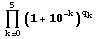-         the pseudo quotientsare in register C, as for logarithms,
-         and we start part 2 with the remainder in register B.

As for logarithms, the pseudo multiplication is done at address 02231 (a + b -> a[w]) and the automaton is driven in this case by the pseudo quotients in C (in the logarithm case it was the result). This simplifies a lot the algorithm, since in the reverse mode, there is no test to make on.

We have however to take into account the remainder r.

We pass from an additive equation: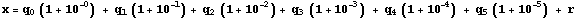to a multiplicative one, and as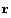is very small we have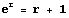and we can wirte :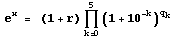To avoid the multiplication by (r +1) and have only shift and add operations, a simple arrangement is made: we’ll add 1 at the leftmost rank of the mantissa a[p] (byte 12), after each pseudo multiplication.

The direct calculation, starting with r = 0.70319 10-6 , gives r + 1 = 1.0000070319 multiplied 2 times by 1.00001 = 1.000027032140638.

Now, computing only on aligned left mantissa (byte 13 reserved for SR count, and byte 12 reserved for this a[p]+1 operation at address 02207 and 02222), the scheme is:

7.0319 + 0.000070319 = 7031970319, then a[p]+1 makes 17.031970319, and once again (q5=2) + 0.0001703197 = 17.0321406387

then operation a[p]+1 makes finally 27.0321406387, divided by 10, at 02213 by a shift right.

The result is the same, but we started directly from the remainder of part 1, and we have the maximum of accuracy.

Here are the results step by step (to help reading the trace) and the flow chart.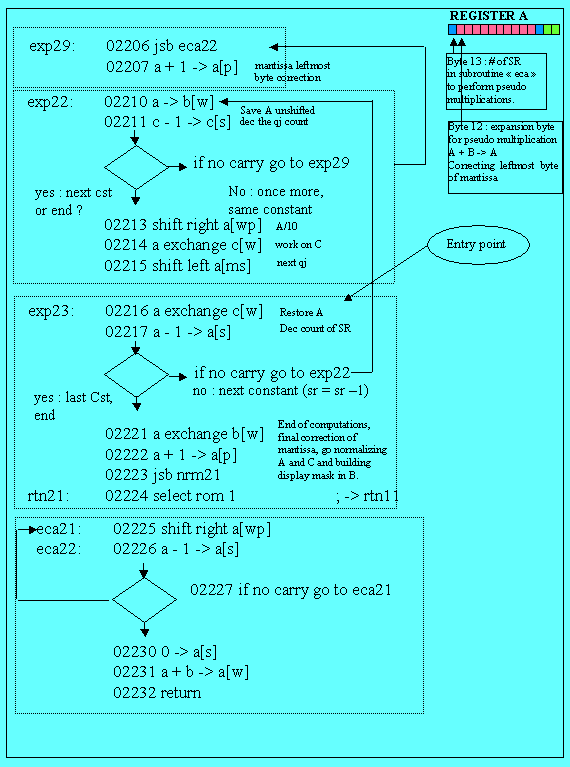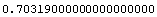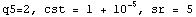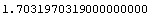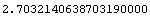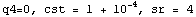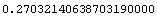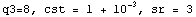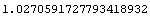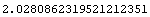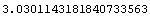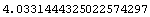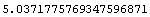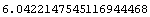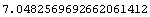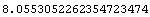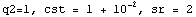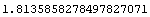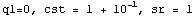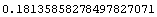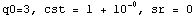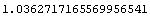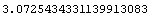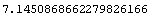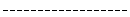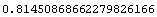In [BP6], the pseudo multiplications cycle is over and the result is register A; it’s time for normalization of registers A and C building floating point exponent and building mask in register B, before going to the display loop waiting for another key.

Normalization (nrm21 …) and mask building (in of13) are not very difficult to guess.

I’ll comment the service routines, in depth, later.

J.L
16 December 2005

_______________

(1) A similar algorithm is given by D. Knuth as an exercise in the Volume 1 of “The Art of Computing programming” (2nd edition) page 26, exercise n° 28; the answer being given p. 469 ; the name of J. E. Meggitt appears in a note. Knuth examines only the binary case.